Next: Summary Up: Examples Previous: Fixed form Q

## Free Form Q

Instead of assuming the form for the approximate posterior distribution we could instead derive the optimal separable distribution (the functions that minimise the cost function subject to the constraint that they be normalised).

Instead of learning the log-std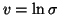, we shall learn the inverse noise variance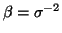. The prior onis assumed to be a Gamma distribution of the form(34)

Setting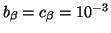leads to a broad prior in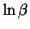, this is equivalent to assuming that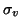is large in the log-std parametrisation.

The cost function that must be minimised is now

 C =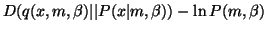=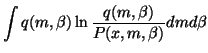(35)

If we assume a separable posterior, that is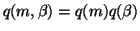and substitute our priors into the cost function we obtain
 C =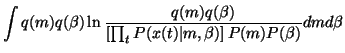=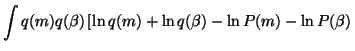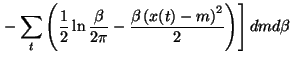(36)

Assuming we know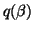we can integrate overin C (dropping any terms that are independent of m) to obtain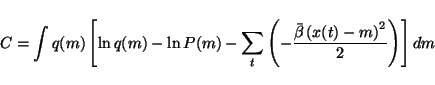(37)

where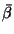is the average value ofunder the distribution. We can optimise the cost function with respect to q(m) by performing a functional derivative.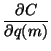=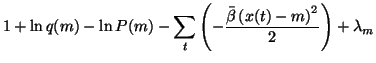= 0 (38)

where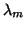is a Lagrange multiplier introduced to ensure that q(m) is normalised. Rearranging we see that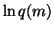=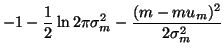=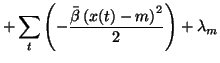(39)

and so the approximate posterior distribution is a Gaussian with variance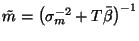and mean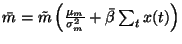.

We can obtain the optimum form forby marginalising the cost function over m and dropping terms independent of.

 C =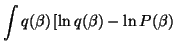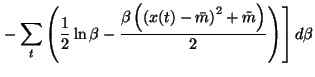(40)

Again we can perform a functional derivative to obtain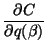=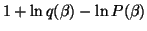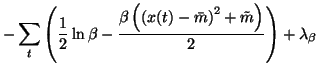= 0 (41)

and so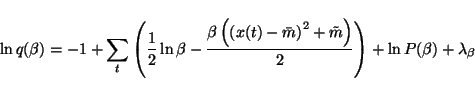(42)

So the optimal posterior distribution is a Gamma distribution, with parameters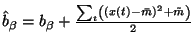and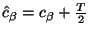. Therefore the expectation ofunder the posterior distribution is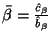.

The optimal distributions for m anddepend on each other (q(m) is a function ofandis a function of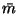and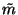) so the optimal solutions can be found by iteratively updating q(m) and.

A general point is that the freeform optimisation of the cost function will typically lead to a set of iterative update equations where each distribution is updated on the basis of the other distributions in the approximation.

We can also see that if the parametrisation of the model is chosen appropriately the optimal separable model has a similar form to the prior model. If the prior distributions are Gaussians the posterior distributions are also Gaussians (likewise for Gamma distributions). If this is the case then we can say that we have chosen conjugate priors.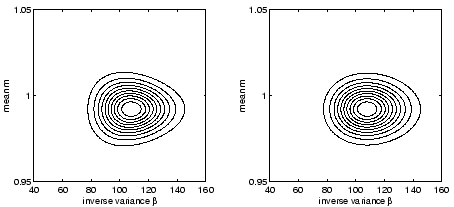Figure 4 shows a comparison of the true posterior distribution and the approximate posterior. The data set is the same as for the fixed form example. The contours in both distributions are centred in the same region, a model that underestimates m. The contours for the two distributions are qualitatively similar, the approximate distribution also shows the assymmetric density.Next: Summary Up: Examples Previous: Fixed form Q
Harri Lappalainen
2000-03-03